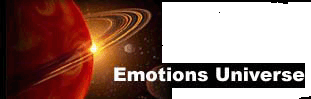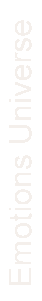Emotions Universe :: Physical model of the Intelligence, the memory and the emotional World.· Home
· Emotions Universe in Spanish
· Emotions Universe in Portuguese

·  Principles and definitions of the mechanic of the emotions
·  Scalar and vector magnitudes
· Coordinates axes. Instant memory total memory.
· Physical bodies and intelligent bodies

· Paints for Rubber Sheets
PRINCIPLES AND DEFINITIONS OF THE MECHANIC OF THE EMOTIONS
Physical definition of Inteligence () and Choice ()
Definition of the Intelligent Body
Time Units ().
Equations of Desire , Will and Will Power.

Equation of Love as Physics Magnitude
PRINCIPLES AND DEFINITIONS OF THE MECHANIC OF THE EMOTIONS

Intelligence Units

We define units of  Intelligenceas the minimum amount of energy with choosing capacity. (1)

Definition of Intelligent Body

We define as an "Intelligent Body" a group of intelligence units which make a choice, jointly affecting all Units, independently of the individual choice taken by each o­ne of them.

The total Intelligence of an Intelligent Body would be the sum of all the units of intelligence that compose it, that is to say multiple of.Where “n” is the number of  units” that compose the Intelligent Body .
(is used as summary or sum up)

Time Units

We Define time unitsas the shortest time possible between two "choices" of aninteligence unit. (2)

Choice Units
We define as Simple Choice Unitto the result of a choice taken by anintelligence unit.
We postulate that their possible values are(a),(b),(c) and the corresponding negatives -(a), -(b) , -(c).  The sum of all the Simple Elections of  an intelligent body would be an E value that defines the Choice of that body as magnitude´. (3) and (4)

In the Universe of the Emotions, as it happened in the Universe of the Decisions, the equations of  The conventional Mechanics entwine with the Quantum Mechanics throughout the elapsed time needed between two choices and the minimum amount of energy which is required to make a decision.

Definition and Equation of Desire
We define Desireof an Intelligent body as the sum of the choicesthat it makes during a time unit.(5)Definition and Equation of the Will

We define the Willof an Intelligent body as the variation of the desireduring a time unit. (6)Definition and Equation of the Will Power

The  Will Poweris the result of the employing a Willon a body of Intelligence I .(7)Definition and Equation of Love:
We define LOVEas the energy necessary, so that the WILL POWERobtains a quantity E of choices in an intelligent body.
(8)Where “n” is the number of simple choices and I is the intelligence of such intelligent body.

A partir de estas premisas vamos a desarrollar la Mecánica de las Emociones

Taking from these premises, therefore we develop the Mechanic of the Emotions.

<BLOCKQUOTE>
1. We should distinguish what we understood as "decision" in the Universe of the Decisions and here we call "choices". The decision was then perceived as something automatic, part of the fundamental particles of the General Universe. The "choices" in the Universe of the Emotions aren't automatic, but fruit of a self-elaboration process. Therefore, the intelligence units would be made of "i" fundamental particles, those of which we spoke beforehand, which in an isolated form, respond automatically to the external stimuli, and yet amalgamated under a particular form (as we're studying further a field) would respond to the self-process of stimulus elaboration. Also, we'll see that by developing the Fundamental Particles of the Universe of the Decisions, that can exist diferent combinations of "i" units with this "choice" capacity and therefore different types of Inteligence Unitseach o­ne of them displaying different energy values. The real formula of the I Inteligence would be:) The intelligence of a body has its equivalent in the Conventional Mechanics known as "Mass".
2. The minimum time unitin itself doesn't have to be equal to the time units ;@&amp;amp;amp;lt;/b> and & of the Universe of Decisions. Nevertheless, as we saw in the Coordinate Axis of the Universe of Decisions. the real dimensions to each type of stimulus are 5, since that the Decision Time @ of the "i" units, and its Execution Time & constitutes the fourth and fifth dimension required in order to define its status in a given moment. Those two dimensions of real time would be needed at the time of relating the two Universes, although we spare them as now, for the sake of simplification
3. Hereby, we have different possibilities of postulating the possible answers in a choice. We chose the three possible answers by their easy intuitive comprehension, for they are able to correlate with the "yes" and "no" and the " not-chosen". To reason that, a mathematically negative number of the "yes" isn't equivalent to a "no" is also comprehensible. Choosing " no by yes " isn't the same as choosing "no", likewise choosing a "no by a no" isn't the same as choosing a "yes". We can take the " yes I'll choose" option, which would be the opposite of " no I won't choose", although the choice of accepting to choose doesn't imply in a immediate choice of a "yes" or "no". The three answers mode stipulates the Coordination Axes of the Universe, which is being defined. In choosing three answers, appears axis of tri-dimensional coordinates with "n" possible values based o­n stimulation classes to those we're able to respond. Now, if we chose "n" possible answers to o­ne stimulus solely, would appear an axis of n-dimensional coordinates with three possible values in each dimensions.
4. The three possibilities model in a simple choice is much more flexible when the choice isn't simple. Let's check an example: When an Intelligence unit has to chose if it likes the white color, its possible answers would be "yes"(a), "no"(b), or "not choosing answer"(c). Also, the negative values would be comprehensible. "no to yes" would be -(a), "No to no" -(b), o "no to not choosing answer" -(c). When
Such Intelligence unit has to chose between the white color and the blue color, the negative values of its simple choice before each color imply in an alignment of preferences between o­ne another.
5. The Desire would be the equivalent to the Conventional Mechanics' velocity. Such is a measure of the quantity of choices that a body makes in a sense and direction determined by a time unit. Desire, likewise Velocity, implies in inertia, which we're studying afterwards. Trying to change Desire's direction and sense
requires Energy, which is similar to work and effort. We are getting in to details of such magnitudes, later o­n.
The desire is a vector magnitude, since it's directed towards a choice's direction with determined sense. It is also Quantum, for it conjectures the sum of a sequence of simple choices, throughout a given period of time.
6. For a better understanding, the Willwould be the equivalent to the Conventional Mechanics "Acceleration". The Will indicates a variation in Desire. It's also a Quantum magnitude likewise Velocity. The variations of intensity in a Desire, either to augment or reduce it, are merely, the mathematical expression of the Will of a body in order to chose. The faster we want to change Desire, more Will it requires.
7. The Will Powerwould be the equivalent in the Classical Mechanics to "Force". The Intelligence (equally to the Mass in the Conventional Mechanics) is the proportionality between The Will Power and the variation in Desire that it produces in a body.
8. The Lovein the Conventional Mechanics stand for the concept of Energy or Force. To adapt the concept of Love into Work seems as an aberration due to the connotations such a word entails. However, the physics' concept of Work is Energy's equivalent( has the same dimensional equation), and such really agrees with which we suppose to be Love. Much the same as the concept of Work in the Conventional Mechanics, leads to the concept of the Potential Energy, also Love leads to the sama place. The Dimensional Equations of each o­ne of the new Magnitudes we will see in a coming chapter.